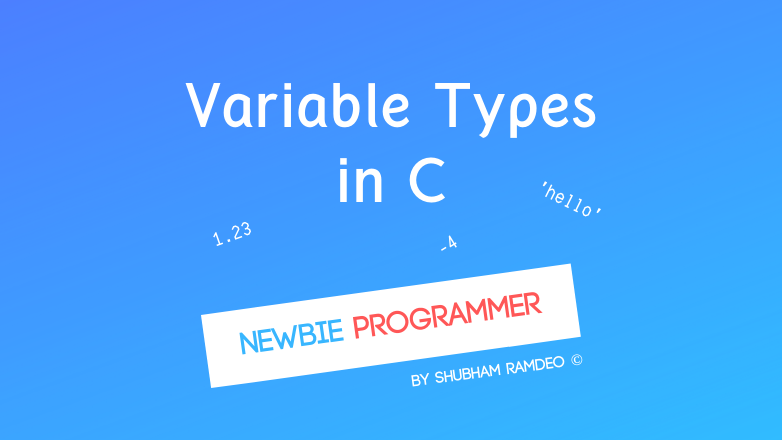# Basic Variable Types in C

PUBLISHED Jul 14th, 2015

What can be put into the variable handbag ? Let’s discuss basic types of variables !Welcome back to the Newbie Programmer Series. In the last part, we have discussed what are variables. In this part, we will discuss the different types of variables. So if you are new to this series, please go to index (here) and real out all the earlier parts so that you can easily understand what I am talking about.

### Why different variable types ?

Based on the application, we have different kinds of information, like numbers, name, date etc. In computer programming, such kinds are called as Data Types. In real life, while handling any information, say a School attendance report, we make a separate column for Roll Numbers, A separate column for Names, A separate column for their attendance etc. We make and keep everything separate, as a Table. But Why ? To keep all the data easy to handle. When you want to search for a name of student, you will just go through the name column. And if you want to find his/her attendance, simply see the corresponding attendance column. That’s so Simple !

Similarly, computer also prepares separate tables in its memories so that it becomes very fast to calculate and process the data.

## Basic Variables types available in C

In C language, we have following Basic types :

#### Integer

There is a little maths and that is :

Integers are simply the “simple complete” numbers like :

0, 1, 2 , 3,…. 6666, -1, -2, -45,…..

All the negative and positive numbers and zero are called integers. These are complete numbers. 1/2,  3.14 etc are not complete numbers, these are fractions and are not integers.

We use the keyword int to make a variable work as an integer.  To print its value, use %d

For an example : This program can be used to calculate area of a square using its formula : area = side x side :

``````#include <stdio.h>
main()
{
int side;
int area;
side = 20;
area = side * side;
printf ("Answer is %d \n", area);
}
``````

The above example first declare that the variables with name ‘side’ and ‘area’ will store some integers. Then ‘side’ is equal to 20. And ‘area’ is equal to ‘side x side’ which means 20 x 20 and so value of ‘area’ become 40. And we printed it.

So Output becomes :

``````Answer is 40
``````

#### Fraction

In the above block, we have seen that integers are complete numbers. But what about the fractional numbers like 99.0243, 1/2, 0.75, 3.1415 etc ? These values can be stored using the keyword float. Its value can be printed using %f

For example : This program can be used to calculate are of a circle using its formula : area = 2 x pi x radius :

``````#include <stdio.h>
main()
{
pi = 3.14;
area = 2 * pi * radius;
printf ("Answer is %f \n", area);
}
``````

The above example declares that radius, pi and area will hold fractional values. Then we assigned the variables to their values and printed.

Note in this example, instead of declaring variables like this :

``````...
float pi;
float area;
...
``````

We saved some space and did like this :

``````...
...
``````

#### Single Character

The above variables contain numbers only. What about alphabets and other symbols ? We can store them using the keyword char and print their values using %c

In numbers, we do like “x = 44”, but we characters, we use the single commas like  ”x = ‘a’”

For example : This is a useless program ! :

``````#include <stdio.h>
main()
{
char letter;
letter = 'S';
printf ("The variable letter is holding the value : %c", letter);
}
``````

In the above example, “letter” is a kind of character variable that is storing ‘S’ letter. Changing its value from ‘S’ to ‘@’ will change the output to “The variable letter is holding the value : @”.

That’s all good but you might be wondering where can I store a complete sentence ? Well there are many more things to discuss but to keep everything simple, I am ending this part here and will discuss more in the next part. Stay Connected :)

#### You might also like:

(prev)
(next)

(rand) Basic Structure of HTML page
(rand) Simple DIY Pickup to record musical instruments
(rand) Logical Operators